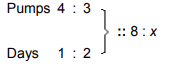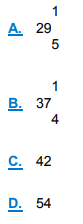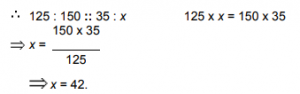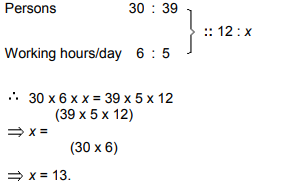## Chain Rule

### Suggested Lessons

1. Direct Proportion: Two quantities are said to be directly proportional, if on the increase (or decrease) of the one, the other increases (or decreases) to the same extent.   Eg. Cost is directly proportional to the number of articles. (More Articles, More Cost) 2. Indirect Proportion: Two quantities are said to be indirectly proportional, if on the increase of the one, the orther decreases to the same extent and vice-versa. Eg. The time taken by a car is covering a certain distance is inversely proportional to the speed of the car. (More speed, Less is the time taken to cover a distance.) Note: In solving problems by chain rule, we compare every item with the term to be found out. 1. 3 pumps, working 8 hours a day, can empty a tank in 2 days. How many hours a day must 4 pumps work to empty the tank in 1 day? A. 9 B. 10 C. 11 D. 12   Answer: Option D Explanation: Let the required number of working hours per day be x. More pumps, Less working hours per day (Indirect Proportion) Less days, More working hours per day (Indirect Proportion)∴ 41x = 328x =x = 12.   2. If the cost of x metres of wire is d rupees, then what is the cost of y metres of wire at the same rate? A. Rs.B. Rs. (xd) C. Rs. (yd) D. Rs.Answer: Option D  Explanation: Cost of x metres = Rs. d. Cost of 1 metre = Rs.Cost of y metres = Rs.= Rs.3. Running at the same constant rate, 6 identical machines can produce a total of 270 bottles per minute. At this rate, how many bottles could 10 such machines produce in 4 minutes? A. 648 B. 1800 C. 2700 D. 10800 Answer: Option B Explanation: Let the required number of bottles be x. More machines, More bottles (Direct Proportion) More minutes, More bottles (Direct Proportion)∴ 61x = 104270x =x = 1800. 4. A fort had provision of food for 150 men for 45 days. After 10 days, 25 men left the fort. The number of days for which the remaining food will last, is:Answer: Option C      Explanation: After 10 days : 150 men had food for 35 days. Suppose 125 men had food for x days. Now, Less men, More days (Indirect Proportion)5. 39 persons can repair a road in 12 days, working 5 hours a day. In how many days will 30 persons, working 6 hours a day, complete the work? A. 10 B. 13 C. 14 D. 15 Answer: Option B Explanation: Let the required number of days be x. Less persons, More days (Indirect Proportion) More working hours per day, Less days (Indirect Proportion)6. A man completesof a job in 10 days. At this rate, how many more days will it takes him to finish the job? A. 5 B. 6 C. 7 D. 7Answer: Option B Explanation: Work done = 5 8 Balance work = 1 - 5 = 3 8 8 Let the required number of days be x. Then, 5 : 3 = :: 10 : x 5 x x = 3 x 10 8 8 8 8 x = 3 x 10 x 8 8 5 x = 6. 7. If a quarter kg of potato costs 60 paise, how many paise will 200 gm cost? A. 48 paise B. 54 paise C. 56 paise D. 72 paise Answer: Option A Explanation: Let the required weight be x kg. Less weight, Less cost (Direct Proportion) 250 : 200 :: 60 : x 250 x x = (200 x 60) x= (200 x 60) 250 x = 48. 8. In a dairy farm, 40 cows eat 40 bags of husk in 40 days. In how many days one cow will eat one bag of husk? A. 1 B. 1 40 C. 40 D. 80 Answer: Option C Explanation: Let the required number of days be x. Less cows, More days (Indirect Proportion) Less bags, Less days (Direct Proportion) Cows 1 : 40 :: 40 : x Bags 40 : 1 1 x 40 x x = 40 x 1 x 40 x = 40. 9. A wheel that has 6 cogs is meshed with a larger wheel of 14 cogs. When the smaller wheel has made 21 revolutions, then the number of revolutions mad by the larger wheel is: A. 4 B. 9 C. 12 D. 49 Answer: Option B Explanation: Let the required number of revolutions made by larger wheel be x. Then, More cogs, Less revolutions (Indirect Proportion) 14 : 6 :: 21 : x 14 x x = 6 x 21 x = 6 x 21 14 x = 9.(Ans) 10. If 7 spiders make 7 webs in 7 days, then 1 spider will make 1 web in how many days? A. 1 B. 7 2 C. 7 D. 49 Answer: Option C Explanation: Let the required number days be x. Less spiders, More days (Indirect Proportion) Less webs, Less days (Direct Proportion) Spiders 1 : 7 :: 7 : x Webs 7 : 1 1 x 7 x x = 7 x 1 x 7 x = 7 11. A flagstaff 17.5 m high casts a shadow of length 40.25 m. The height of the building, which casts a shadow of length 28.75 m under similar conditions will be: A. 10 m B. 12.5 m C. 17.5 m D. 21.25 m Answer: Option B Explanation: Let the height of the building x metres. Less lengthy shadow, Less in the height (Direct Proportion) 40.25 : 28.75 :: 17.5 : x 40.25 x x = 28.75 x 17.5 x = 28.75 x 17.5 40.25 x = 12.5 12. In a camp, there is a meal for 120 men or 200 children. If 150 children have taken the meal, how many men will be catered to with remaining meal? A. 20 B. 30 C. 40 D. 50 Answer: Option B Explanation: There is a meal for 200 children. 150 children have taken the meal. Remaining meal is to be catered to 50 children. Now, 200 children 120 men. 50 children 120 x 50 = 30 men. 200 13. An industrial loom weaves 0.128 metres of cloth every second. Approximately, how many seconds will it take for the loom to weave 25 metres of cloth? A. 178 B. 195 C. 204 D. 488 Answer: Option B Explanation: Le the required time be x seconds. More metres, More time (Direct Proportion) 0.128 : 25 :: 1 : x 0.128x = 25 x 1 x = 25 = 25 x 1000 0.128 128 x = 195.31. Required time = 195 sec (approximately). 14. 36 men can complete a piece of work in 18 days. In how many days will 27 men complete the same work? A. 12 B. 18 C. 22 D. 24 E. None of these Answer: Option D Explanation: Let the required number of days be x. Less men, More days (Indirect Proportion) 27 : 36 :: 18 : x 27 x x = 36 x 18 x = 36 x 18 27 x = 24. 15. 4 mat-weavers can weave 4 mats in 4 days. At the same rate, how many mats would be woven by 8 mat-weavers in 8 days? A. 4 B. 8 C. 12 D. 16 Answer: Option D Explanation: Let the required number of bottles be x. More weavers, More mats (Direct Proportion) More days, More mats (Direct Proportion) Wavers 4 : 8 :: 4 : x Days 4 : 8 4 x 4 x x = 8 x 8 x 4 x = (8 x 8 x 4) (4 x 4) x = 16.